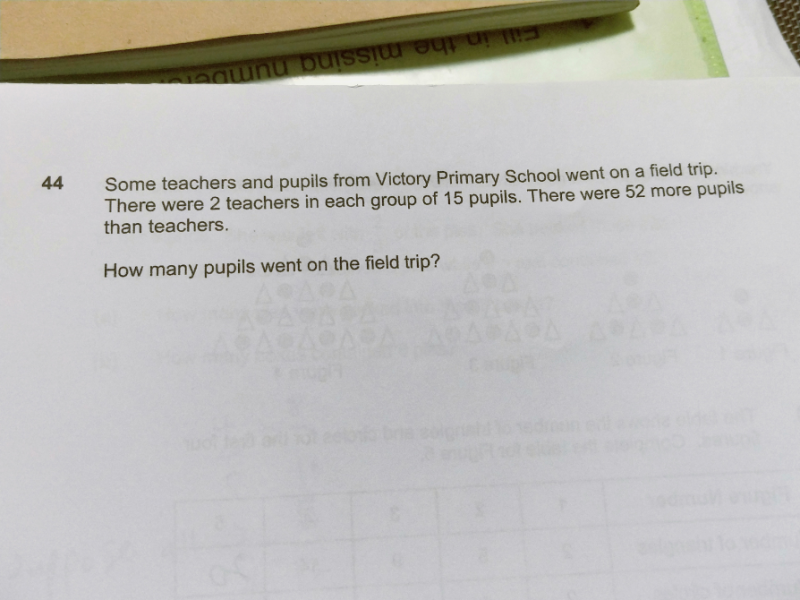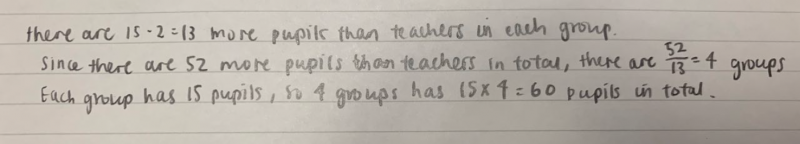# QuestionHi guys need help with this question. TIA!

In every group, there are 15 pupils and 2 teachers. This means that there are (15 – 2 = 13) more pupils than teachers in every group.

Since there are 52 more pupils than teachers in total, this means that the total difference between the pupils and teachers across all the groups will add up to 52. Since each group has a difference of 13 more pupils than teachers, we can find the number of groups by (52 ÷ 13 = 4) groups.

Since each group has 15 pupils, the total number of pupils will be (15 x 4 = 60 pupils)

 # 0f group(s) # of pupils # of teachers Difference 1 15 2 13 2 30 4 26 3 45 6 39 4 60 8 52

0 Replies 0 Likes ✔Accepted Answer0 Replies 2 Likes